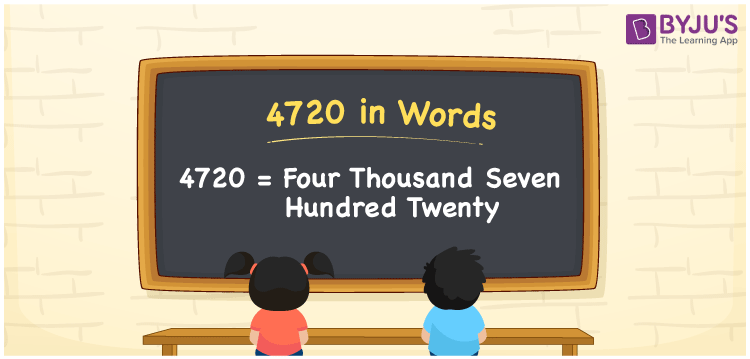# 4720 in Words

4720 in words is written as “Four Thousand Seven Hundred Twenty”. In Maths, 4720 is a cardinal number that expresses a quantity or a value. For example, the price of a Bluetooth speaker is Rs.4720 or Rupees Four Thousand Seven Hundred Twenty. Learn more about Numbers In Words at BYJU’S.

 4720 in Words Four Thousand Seven Hundred Twenty Four Thousand Seven Hundred Twenty in Numbers 4720

## 4720 in English Words## How to Write 4720 in Words?

The number 4720 in words can be written using a place value chart, where we can identify the position of each digit. Since 4720 is a four-digit number, thus the place value of the digits is given by:

 Thousands Hundreds Tens Ones 4 7 2 0

From the above table,

4 → Thousands place

7 → Hundreds place

2 → Tens

0 → Ones place

Hence, when we read the number from right to left, it is Four Thousand Seven Hundred Twenty.

### Expanded Form of 4720

We can write the expanded form as:

4 x Thousand + 7 × Hundred + 2 × Ten + 0 × One

= 4 x 1000 + 7 × 100 + 2 × 10 + 0 × 1

= 4000 + 700 + 20 + 0

= Four Thousand Seven Hundred Twenty

4720 is a whole number that is succeeded by 4719 and preceded by 4721. Learn more about the number 4720 below:

• 4720 in Words – Four Thousand Seven Hundred Twenty
• Is 4720 an odd number? – No
• Is 4720 an even number? – Yes
• Is 4720 a perfect square number? – No
• Is 4720 a perfect cube number? – No
• Is 4720 a prime number? – No
• Is 4720 a composite number? – Yes

## Frequently Asked Questions on 4720 in words

Q1

### What is 4720 in words?

4720 in words is given by Four Thousand Seven Hundred Twenty.
Q2

### What is the rule to write 4720 in words?

4720 in words is written based on the place values of each digit. 4 is at thousands, 7 at hundreds, 2 at tens and 0 at ones.

4 → Thousands place

7 → Hundreds place

2 → Tens

0 → Ones place

Thus, reading from right to left, it is Four Thousand Seven Hundred Twenty.

Q3

### What is the value of 4720 + 100 in words?

4720 + 100 = 4820, i.e., Four Thousand Eight Hundred Twenty in words